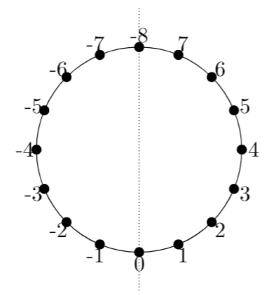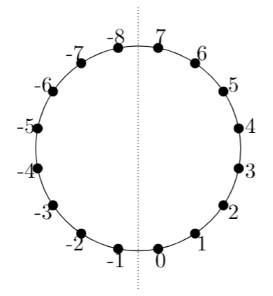## Wednesday, 3 October 2018

### abs and its "extra" result

The abs function has, in its usual (most useful) formulation, one extra value in its codomain than just "all non-negative values". That extra value is the most negative integer, which satisfies abs(x) == x despite being negative. Even accepting that the absolute value of the most negative integer is itself, it may still seem strange (for an operation that is supposed to have such a nice symmetry) that the size of the codomain is not exactly half of the size of the domain.

That there is an "extra" value in the codomain, and that it is specifically the most negative integer, may be more intuitively obvious when the action of abs on the number line circle is depicted as "folding" the circle symmetrically in half across the center and through zero (around which abs is supposed to be symmetric), folding the negative numbers onto the corresponding positive numbers:Clearly both zero and the most negative integer (which is also on the "folding line") stay in place in such a folding operation and remain part of the resulting half-circle. That there is an "extra" value in the codomain is the usual fencepost effect: the resulting half-circle is half the size of the original circle in some sense, but the "folding line" cuts through two points that have now become endpoints.

By the way the "ones' complement alternative" to the usual abs, let's call it OnesAbs(x) = x < 0 ? ~x : x (there is a nice branch-free formulation too) does have a codomain with a size exactly half of the size of its domain. The possible results are exactly the non-negative values. It has to pay for that by, well, not being the usual abs. The "folding line" for OnesAbs runs between points, avoiding the fencepost issue: